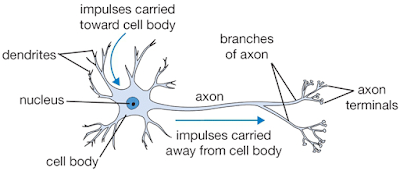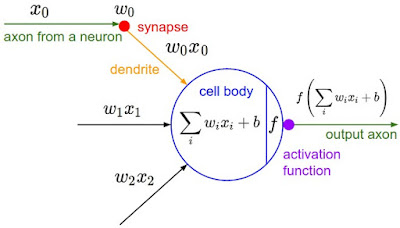## Friday, September 08, 2017

### Neural Network with R: A concept 

A post shared by Bagus Tris Atmaja (@bagustris) on

Neural network is one of the earlier and most developed artificial intelligence. The purpose of the computer, i.e., internet is to differ the picture between cats and dog, or between camel and dromedary.  The human brain is very good at recognising which is which. We can easily detect if the shown picture is camel of dromedary. But, how about computer? Can computer distinguish the image of camel and dromedary? Here is how the neural net works.

Real Neuron Vs Artificial Neuron
On the real human brain, each neuron takes information from other neurons, processes them, and then produces an output. One could imagine that certain neurons output information based on raw sensory inputs, other neurons build higher representations on that, and so on until one gets outputs that are significant at a higher level .Real neuron architecture, source 

On the artificial neuron, the single artificial neuron will do a dot product between w and x, then add a bias, the result is passed to an activation function that will add some non-linearity. The neural network will be formed by those artificial neurons.

The non-linearity will allow different variations of an object of the same class to be learned separately. Which is a different behaviour compared to the linear classifier that tries to learn all different variations of the same class on a single set of weights. More neurons and more layers is always better but it will need more data to train.+

Each layer learn a concept, from it's previous layer. So it's better to have deeper neural networks than a wide one .Artificial neuron,

Classification
We, human being, can recognise and any objects:

• Quickly
• Robustly
• Reliably

and we, human being, don’t use conventional logic, i.e. flow charts, we just think. In similar cases, this attacks a very general statistics/data problem:
• Physicist: is this event signal or background is the track a muon or a pion?
• Astrnomer: is this blob a star or a galaxy?
• Doctor: is this patient sick or well?
• Banker: is this company a sound investment or junk?
• Employer: is this applicant employable or a liability?

Neural Networks
The human brain is made of ~100,000,000,000 neurons. Each neuron has MANY inputs. From external sources (eyes, ears...) or from other neurons. Each neuron has one output connected to MANY externals (muscles or other neurons). The neuron forms a function of the inputs and presents it to all the outputs.

Artificial Neural Networks (ANN) is a try to replicate human neural networks in a software. Neuron is represented by node, which has many inputs, $U_j$, weighted with $w_{ij}$, so then the value entering neuron form, $y_i=\Sigma w_{ij} U_j$. The generated output is

$$U_i = F(y_i) = F( \Sigma w_{ij} U_j)$$

F is thresholding function which gives monoton output around center area and saturated ouput at top and bottom extreme area. Sigmoid and $\tanh$ are often used as thresholding function on basic ANN.

$$F(y) = \dfrac{1}{1+exp^{-y}}$$

For $\tanh$ function
$$F(y) = \tanh(y)$$

The function can be shown below,

The Multi Layer Perceptron
A system for binary classification: recognise data ‘events’ (all of the same format) as belonging to one of 2 classes. e.g. signal and background, S and B.

Nodes arranged in layers. First layer – input, Last layer –single output, ideally 1 (for S) or 0 (for B).
In between - ‘hidden’ layers
Action is sychronised: all of first layer effects the second (effectively) simultaneously, then second layer effects third, etc

How do we set the weights?
The goal of ANN is how to set the weight so the given input match the output. We use training data:use samples of known events.

Present events whose classification is known: has a desired output T, which is 0 or 1. Call the actual output U.

Define ‘Badness’,  $B= \dfrac{1}{2} (U-T)^2$. “Training the net” means adjusting the weights to reduce total or average B.

Strategy: change each weight $w_{ij}$ by step proportional to $-dB/dw_{ij}$ .

Do this event by event (or in batches, for efficiency). All we need to do is calculate those differentials, start with final layer and work backwards ('back-propagation').

To be continued on computation/programming side.

Reference:
 Artificial Neural Networks (Roger Barlow)
 https://rstudio-pubs-static.s3.amazonaws.com/146706_0754aef7cdab424ebe0ac4e0e5aa362e.html
 https://leonardoaraujosantos.gitbooks.io/artificial-inteligence/neural_networks.html